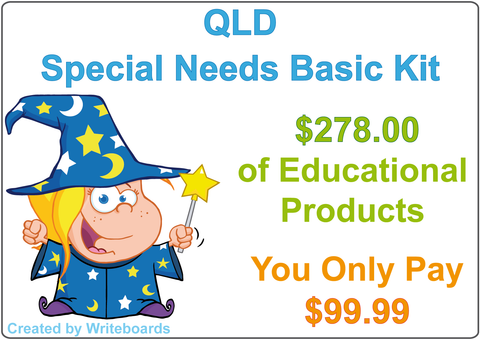# Basic Maths Pack - QLD Modern Cursive Font

QLD Modern Cursive Font - Basic Maths Pack

• This pack includes addition, subtraction, division and multiplication worksheets from one to twelve.
• All our maths worksheets have been completed using QLD Modern Cursive Font handwriting.

These QLD Modern Cursive Font maths worksheets are completed in order and come both with and without the answers.

• Maths Subtraction one to four, Maths Subtraction five to eight, Maths Subtraction nine to twelve.
• Maths Division one to four, Maths Division five to eight, and Maths Division nine to twelve.
• Times Tables -  one to four, five to eight and nine to twelve.
These QLD Modern Cursive Font maths worksheets are completed using a mixed up order and come both with and without the answers.
• Addition one to four, five to eight and nine to twelve.
• Subtraction one to four, five to eight and nine to twelve.
• Division one to four, five to eight and nine to twelve.

NOTE: This download is for HOME USE only. If you are a school, teacher, business or professional person you will require a Writeboards Site Licence.

#### Related Products for Parents: